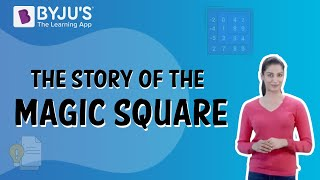Jet Set Go! All about Aeroplanes Jet Set Go! All about Aeroplanes

# Magic Square

One of the types of mathematics called recreational mathematics is brought out for recreation. It means it is designed for entertainment rather than rigorous study and application-based professional activity or part of a student’s formal education. Although it is not necessarily defined as an attempt for learners, many topics in this field do not require an understanding of advanced mathematics. This field of mathematics contains maths puzzles and games, such as magic squares that are often attractive to kids. Also, it helps in motivating their further study of the subject. In this article, you will learn the definition of magic squares in maths, how to create magic squares, uses and tricks of magic squares in detail.

## What is a Magic Square Puzzle?

A magic square is defined as a square containing several distinct integers arranged so that the total or sum of the numbers is the same in every row, column, and main diagonal and usually in some or all of the other diagonals.

Learn: Square

## Magic Square Formula

A magic square puzzle of the order n is an organization of n2 numbers, usually unique integers, in a square. The n numbers in all rows, columns, and diagonals add up to the same constant. A magic square bears the integers from 1 to n2. The fixed sum in every row, column and diagonal is known as the magic constant or the magic sum. It is represented by the letter M. The magic constant of a typical magic square depends entirely on the value of n. Thus, the value of the magic sum is calculated using the formula:

M = n(n2 + 1)/2

This is the formula for a magic square that is used to make magic squares of different orders. If we subtract each number from (n2 + 1), we get another magic square, and this is called the complementary magic square. A square containing consecutive numbers beginning with 1 is often called the normal magic square.

Let’s learn How to make a Magic square of order 3, i.e., 3×3 magic square here along with an example.

### How to Solve Magic square

As mentioned above, the formula of the magic square sum is n(n2 + 1)/2.

For a magic square of order 3, we need to substitute n = 3 to know the magic sum so that we can easily form the magic square 3×3.

When n = 3, the sum = 3(32 + 1) = 3(9 + 1)/2 = (3 × 10)/2 = 15

Now, we have to place the numbers in the respective places so that the sum of numbers in each row, column and diagonal is equal to 15.

Magic Square Trick for order 3

Let “x” be the order of the magic square.

In this case, x = 3.

Consider another number, “y” such that the product of x and y is equal to the magic sum, i.e. 15.

So, y = 5 {xy = (3)(5) = 15}

The value of y should always be at the center of the square and x be on its left cell.

The cell above x is taken as y – 1 as given below: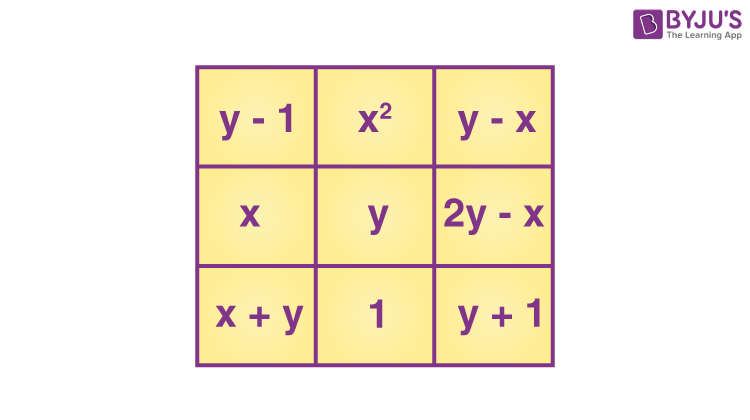Based on this trick, we can build the magic square of order 3.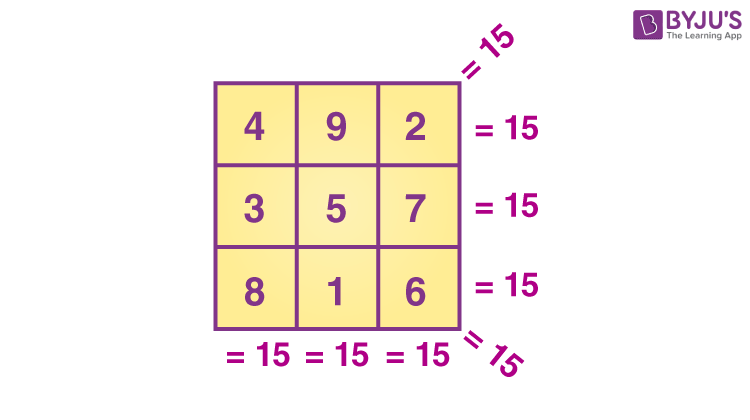## Magic Square 3×3

From the magic square obtained above, we can make multiple magic squares of the same order by changing the numbers in suitable places. Consider the Magic square 3×3 sum 15 given below: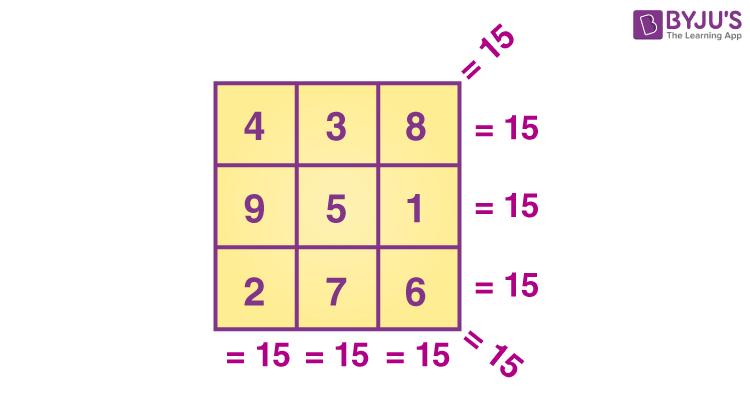Let us make the complementary magic square of the above square.

(n2 + 1) = 32 + 1 = 9 + 1 = 10

Now, subtract each number from (n2 + 1), i.e. from 10.

First row numbers:

10 – 4 = 6

10 – 3 = 7

10 – 8 = 2

Second row numbers:

10 – 9 = 1

10 – 5 = 5

10 – 1 = 9

Third row numbers:

10 – 2 = 8

10 – 7 = 3

10 – 6 = 4

Therefore, the new magic square formed is: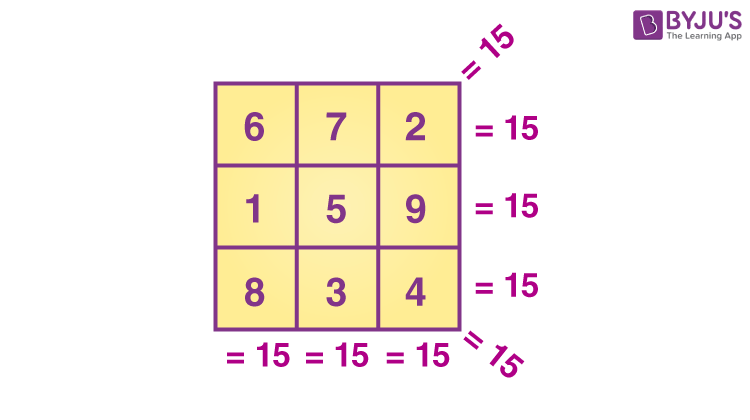### Magic Square 4×4

For a magic square of order 4, we need to substitute n = 4 to know the magic sum so that we can easily form the magic square 3×3.

When n = 4, the sum = 4(42 + 1) = 4(16 + 1)/2 = (4 × 17)/2 = 34

Thus, the magic square 3×3 sum 34 is given as: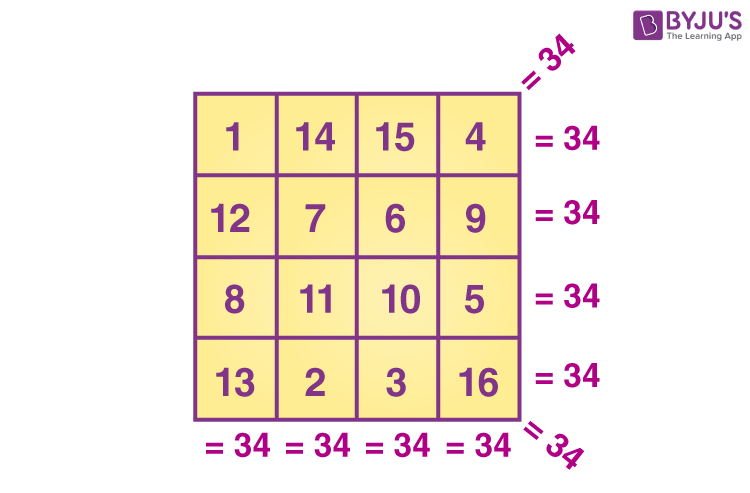We can also make the complementary square of this 4x4x magic square by subtracting each number from (n2 + 1), i.e., from 17.

#### Watch The Below Video To Know The History of Magic Square